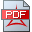Disclaimer: this homepage is no longer maintained; nevertheless, it contains or refers to corresponding relevant papers, which were developed during the last years back to 2011 addressing the three Millennium problems: RH, NSE, YME.

This journey ended up into a combined proposed mathematical solution framework. It is about common Hilbert space based solution, avoiding e.g. gauge principles, co-variant derivatives resp. differentiable manifolds concepts and force type dependent quantum/quark types.

An application of the proposed distributional Hilbert space framework is provided in the context of the non-linear Landau damping phenomenon in plasma physics, where the standard mathematical models are built on; more especially, this is about the Landau, Vlasov, Poisson, Lorentz, Boltzmann PDE systems, which are purely classical PDE systems and the corresponding existing proofs of the Landau damping phenomenon for the linear and the nonlinear cases, are applying classical techniques. In other words, an observed physical quantum mechanics/dynamics phenomenon is proven based on classical mathematical models and related proof techniques.

In section 1 of the following paper a high level overview regarding the newly proposed quantum ("particles": bosons & fermions) state and quantum energy model is provided. It is basically about a common distributional Hilbert space based model for quantum state & quantum mass/energy PDE systems. It enable a "fermions quantum state" Hilbert space H(0), which is dense in H(-1/2) with respect to the H(-1/2) norm, and its related (orthogonal) "bosons quantum state" Hilbert space H(-1/2)-H(0), which is a closed subspace of H(-1/2).Braun K., 3D-NSE, YME, GUT solutionsBraun K., A distributional Hilbert space framework to prove the Landau damping phenomenon

In the following some related papers, which are also provided in navier-stokes-equations.com

Some further paper are provided in section "quantum gravity modelling".

In the following some related papers in the context of a quantum gravity modelling are providedDirac P., The Quantum Theory of the Emission and Absorption of Radiation